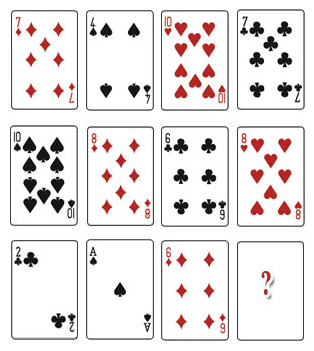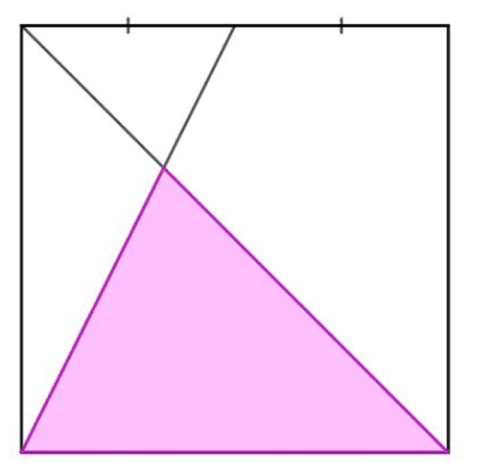Thread Rating:

## Poll

 I love math!10 votes (43.47%) Math is great.9 votes (39.13%) My religion is mathology.5 votes (21.73%) Women didn't speak to me until I was 30.2 votes (8.69%) Total eclipse reminder -- 04/08/20247 votes (30.43%) I steal cutlery from restaurants.3 votes (13.04%) I should just say what's on my mind.4 votes (17.39%) Who makes up these awful names for pandas?3 votes (13.04%) I like to touch my face.9 votes (39.13%) Pork chops and apple sauce.7 votes (30.43%)

23 members have voted

GialmereJoined: Nov 26, 2018
• Threads: 39
• Posts: 1821
May 4th, 2020 at 6:39:42 PM permalinkWhat playing card completes the puzzle?
Have you tried 22 tonight? I said 22.
GialmereJoined: Nov 26, 2018
• Threads: 39
• Posts: 1821
May 6th, 2020 at 6:34:44 AM permalink
Only consider rows.

___________________________

Meanwhile,

Using the numbers 2, 3, 4, and 5, and the symbols + and =, write a true statement. You must use each number and symbol once and only once.
Have you tried 22 tonight? I said 22.
CrystalMathJoined: May 10, 2011
• Threads: 8
• Posts: 1881
Thanks for this post from:May 6th, 2020 at 7:14:30 AM permalink
Quote: Gialmere

Only consider rows.

___________________________

Meanwhile,

Using the numbers 2, 3, 4, and 5, and the symbols + and =, write a true statement. You must use each number and symbol once and only once.

32 = 4 + 5
or
24 + 3 = 5
I heart Crystal Math.
GialmereJoined: Nov 26, 2018
• Threads: 39
• Posts: 1821
May 6th, 2020 at 7:26:04 AM permalink
Quote: CrystalMath

Quote: Gialmere

Only consider rows.

___________________________

Meanwhile,

Using the numbers 2, 3, 4, and 5, and the symbols + and =, write a true statement. You must use each number and symbol once and only once.

32 = 4 + 5
or
24 + 3 = 5

Correct!

I always liked that little trick.

Q) What do you call ginger cut into a cube?

A) A square root.
Have you tried 22 tonight? I said 22.
GialmereJoined: Nov 26, 2018
• Threads: 39
• Posts: 1821
May 6th, 2020 at 3:53:15 PM permalink
Here's another one that made a stir a while back. Hopefully it hasn't already been posted.What fraction of the square is shaded?
Have you tried 22 tonight? I said 22.
ThatDonGuyJoined: Jun 22, 2011
• Threads: 97
• Posts: 4629
Thanks for this post from:May 6th, 2020 at 4:54:53 PM permalink
Quote: Gialmere

Here's another one that made a stir a while back. Hopefully it hasn't already been posted.What fraction of the square is shaded?

I tried to post an image to Imgur, but apparently you can't link directly from Imgur any more.
Anyway, I'll try to describe it...
Let ABCD be the square, with A at the upper left and B at the upper right
Let M be the midpoint of AB
Let X be where AC intersects BM; the shaded triangle is DXC
Let N be the midpoint of CD
Let E and F be on AB and CD, respectively, such that EF goes through X
Assume AB = 2; AM = 1
Let h be the length of EX; FX = 2 - h
MDN is similar to XDF, so XF/FD = MN/ND = 2, and FX = 1 - h/2
ABC is similar to AEX, so AE/EX = AB/BC = 1, which means AE = EX = h, which means FD = h
h = 1 - h/2, so h = 2/3, and 2 - h = 4/3
The area of CXD = 1/2 * (2 - h) * 2 = 4/3
The area of the square is 4, so the fraction = (4/3) / 4 = 1/3

GialmereJoined: Nov 26, 2018
• Threads: 39
• Posts: 1821
May 6th, 2020 at 5:14:53 PM permalink
Quote: ThatDonGuy

I tried to post an image to Imgur, but apparently you can't link directly from Imgur any more.
Anyway, I'll try to describe it...
Let ABCD be the square, with A at the upper left and B at the upper right
Let M be the midpoint of AB
Let X be where AC intersects BM; the shaded triangle is DXC
Let N be the midpoint of CD
Let E and F be on AB and CD, respectively, such that EF goes through X
Assume AB = 2; AM = 1
Let h be the length of EX; FX = 2 - h
MDN is similar to XDF, so XF/FD = MN/ND = 2, and FX = 1 - h/2
ABC is similar to AEX, so AE/EX = AB/BC = 1, which means AE = EX = h, which means FD = h
h = 1 - h/2, so h = 2/3, and 2 - h = 4/3
The area of CXD = 1/2 * (2 - h) * 2 = 4/3
The area of the square is 4, so the fraction = (4/3) / 4 = 1/3

Correct!

Another quick solve for TDG!
-------

Q) How do triangles communicate?

A) They use sine language.
Have you tried 22 tonight? I said 22.
rsactuaryJoined: Sep 6, 2014
• Threads: 21
• Posts: 1795
May 6th, 2020 at 6:36:56 PM permalink
I didn't see a solution to the card puzzle? I can't figure it out.
ssho88Joined: Oct 16, 2011
• Threads: 45
• Posts: 502
Thanks for this post from:May 6th, 2020 at 8:15:45 PM permalink
Quote: Gialmere

Here's another one that made a stir a while back. Hopefully it hasn't already been posted.What fraction of the square is shaded?

Length of square =1,
Equation of slanting line 1 : y = 2x
Equation of slanting line 2 : y = -x + 1

Find Intersection point :-
2x = -x +1
x = 1/3, y = 2/3

Shaded area = 2/3 * 1 * 1/2 = 1/3
Square area = 1

Fraction = 1/3 / 1 = 1/3

GialmereJoined: Nov 26, 2018
• Threads: 39
• Posts: 1821
May 7th, 2020 at 5:58:43 AM permalink
Quote: ssho8

Length of square =1,
Equation of slanting line 1 : y = 2x
Equation of slanting line 2 : y = -x + 1

Find Intersection point :-
2x = -x +1
x = 1/3, y = 2/3

Shaded area = 2/3 * 1 * 1/2 = 1/3
Square area = 1

Fraction = 1/3 / 1 = 1/3

Correct!

Your logic is easier to follow.
Have you tried 22 tonight? I said 22.

• Jump to: